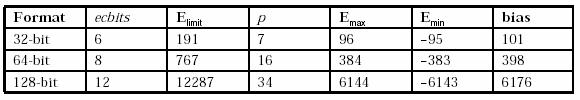# APPENDIX C – Calculation of Emax and bias

This section describes how Emax and Emin (the maximum and minimum exponents available in a format) and bias (the amount to be subtracted from the encoded exponent to form the exponent’s value) are calculated. Except for the calculation of Elimit, these calculations are general for any format where the coefficient and exponent are both integers.

1. Let p be the precision (total length of the coefficient) of a format, in digits.

2. Let Emax be the maximum positive exponent for a value in a format, as defined in IEEE 854. That is, the largest possible number is (10p-1) / (10(p–1)) × 10Emax

For example, if p=7 this is 9.999999 × 10Emax.

3. The exponent needed for the largest possible number is Emax–(p–1) (because, for example, the largest coefficient when p=7 is 9999999, and this only needs to be multiplied by

10Emax / 10(p–1) to give the largest possible number.

4. Emin=–Emax (as defined by IEEE 854 for base 10 numbers). That is, the smallest normal number is 10Emin. The exponent needed for this number is Emin (its coefficient will be 1).

5. The number of exponents, Enormals, used for the normal numbers is therefore 2 × Emaxp + 2. (The values –Emax through –1, 0, and 1 through Emax–(p–1).)

6. Let Etiny be the exponent of the smallest possible (tiniest, non-zero) subnormal number when expressed as a power of ten. This is Emin – (p–1). For example. if p=7 again, the smallest subnormal is 0.000001 × 10Emin, which is 10Etiny.

The number of exponents needed for the subnormal numbers, Esubnormals, is therefore Emin – Etiny, which is p – 1.

7. Let Erange be the number of exponents needed for both the normal and the subnormal numbers; that is, Enormals + Esubnormals. This is (2 × Emax + 1).

8. Place Etiny so its encoded exponent (the exponent with bias added) is 0 (the encoded exponent cannot be less than 0, and we want an all-zeros number to be valid – hence an encoded exponent of 0 must be valid).

9. Let Elimit be the maximum encoded exponent value available. For the formats in the specification, this is 3 × 2ecbits – 1, where ecbits is the length of the exponent continuation in bits (for example, Elimit is 191 for the 32-bit format).

10. Then, the number of exponent values available is Elimit + 1, which is 3 × 2ecbits.

11. Now, to maximize Emax, Erange = Elimit + 1

That is, 2 × Emax + 1 = 3 × 2ecbits.

12. Hence: Emax = (3 × 2ecbits – 1)/2 = Elimit/2

Note that the divisions by 2 must be truncating integer division.

13. If Elimit is odd (always the case in these encodings), one value of exponent would be unused. To make full use of the values available, Emin remains as the value just calculated, negated, and Emax is increased by one.7

Hence: Emin = –Elimit/2

and: Emax = Elimit/2 + 1 (where the divisions by 2 are truncating integer division).

14. And: bias = –Etiny = –Emin + p – 1

For example, let Elimit = 191 and p = 7 (the 32-bit format). Then:

Emax = 191/2 + 1 = 96

Emin = –95

Etiny = –101

bias = 101

The parameters and derived values for all three formats are as follows:Note that it is also possible to consider the coefficients in these formats to have a decimal point after the first digit (instead of after the last digit). With this view, the bit patterns for the layouts are identical, but the bias would be decreased by p–1, resulting in the same value for a given number.